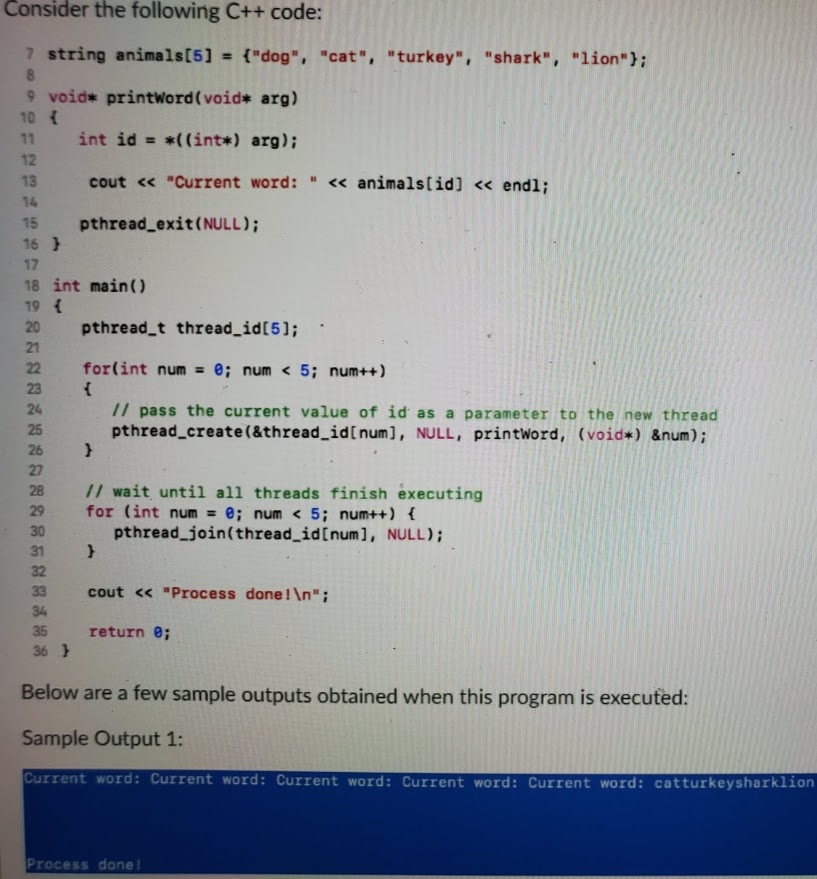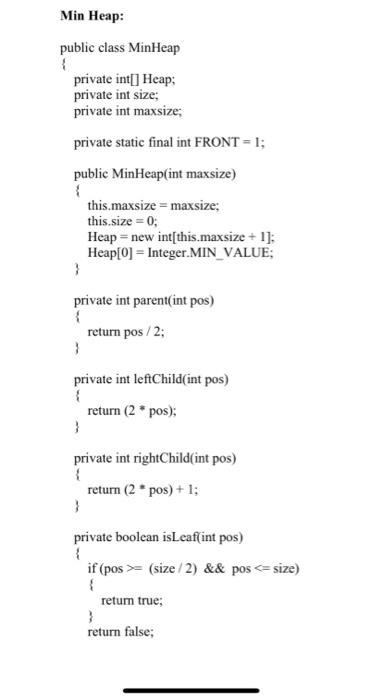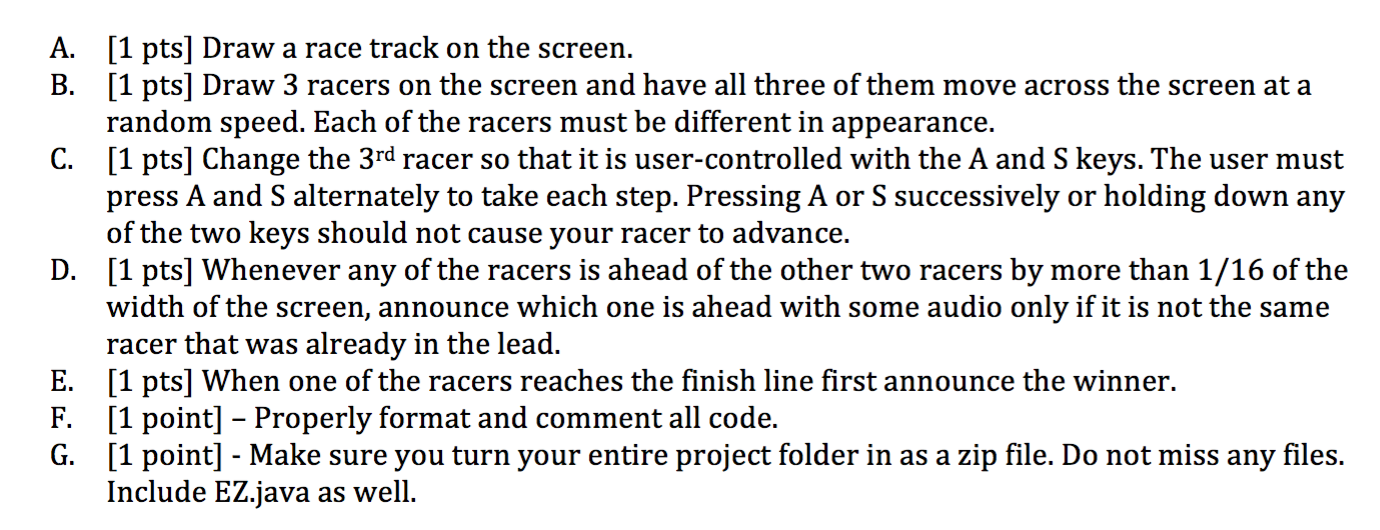Question

1. What is the output?

System.out.print(3 + 3 * 3);

 a. 18 b. 12 c. 9 d. 0 e. 10

2.   What is output by the code below?

System.out.print("\\dog\\cat");

 a. dog b. dogcat c. \\dog\\cat d. \dog\cat e. catdog\\\\

3.   What is returned by the call     getIt(9) ?

public static int getIt(int num){

int ans = 0;

if( num >=2 )

{

if( num >= 7)

ans += 2;

else

ans += 3;

}

ans += 4;

return ans;

}

 a. 4 b. 2 c. 6 d. 7 e. 9

4.   What is returned by the call     getIt(3) ?

public static int getIt(int num)

{

int ans = 0;

if( num >=2 )

{

if( num >= 7)

ans += 2;

else

ans += 3;

}

ans += 4;

return ans;

}

 a. 4 b. 6 c. 7 d. 4 e. 9

5.   What is returned by the call     getIt(1) ?

public static int getIt(int num)

{

int ans = 0;

if( num >=2 )

{

if( num >= 7)

ans += 2;

}

else

{

ans += 3;

}

ans += 4;

return ans;

}

 a. 4 b. 5 c. 6 d. 7 e. 9

6.   What is output by the code below?

//client code

int[] list = {2,12,11,45,52,36,5,3,1};

System.out.println( go(list) );

//go receives an array and returns a calculated value

public static double go( int[] ray )

{

int val = 0;

for(int i=0; i < ray.length; i = i + 2)

val = val + ray[i];

return val;

}

 a. 45 b. 96 c. 56 d. 65 e. 71

7.   What is output by the code below?

//client code

int[] list = {2,12,11,45,52,36};

System.out.println( go(list) );

//go receives an array and returns a calculated value

public static int go( int[] ray )

{

int val = Integer.MIN_VALUE;

for(int i=0; i < ray.length; i++)

if( ray[i] > val )

val = ray[i];

return val;

}

 a. 45 b. 2 c. 12 d. 52 e. 36

8.   What is the code shown below working to locate?

public static int go( int[] ray )

{

int val = Integer.MIN_VALUE;

for(int i=0; i < ray.length; i++)

if( ray[i] > val )

val = ray[i];

return val;

}

 a. The code is trying to locate all of the even numbers. b. The code is trying to locate the biggest number. c. The code is trying to locate the smallest number. d. The code is trying to locate all of the odd numbers. e. The code is trying to locate the biggest even number.

9.   What is output by the code below?

//client code

int[] list = {3, 6, 9, 2, 4, 5};

System.out.println( go(list) );

//go receives an array and returns a calculated value

public static int go( int[] ray )

{

int val = 0;

for(int i=0; i < ray.length; i++)

val = val + ray[i];

return val;

}

 a. 29 b. 27 c. 20 d. 26 e. 25

10.   What is output by the code below?

System.out.println(Math.pow(2,4));

 a. 32 b. 16 c. 16 d. 32 e. 8

QUESTION (1):-

OPTION (B) 12

QUESTION (2):-

OPTION (D) \dog\cat

QUESTION (3):-

OPTION (C) 6

QUESTION (4):-

OPTION (E) 71.0

QUESTION (5):-

OPTION (D) 52

QUESTION (6):-

OPTION (B)

QUESTION (7):-

OPTION (A) 29

QUESTION (8):-

OPTION (C) 16.0

#### Earn Coins

Coins can be redeemed for fabulous gifts.

Similar Homework Help Questions
• ### 1. What is the output when you run printIn()? public static void main(String[] args) { if...

1. What is the output when you run printIn()? public static void main(String[] args) { if (true) { int num = 1; if (num > 0) { num++; } } int num = 1; addOne(num); num = num - 1 System.out.println(num); } public void addOne(int num) { num = num + 1; } 2. When creating an array for primitive data types, the default values are: a. Numeric type b. Char type c. Boolean type d. String type e. Float...

• ### I have this code but when there's 0 pennies, it doesn't output "and 0 pennies" at...

I have this code but when there's 0 pennies, it doesn't output "and 0 pennies" at all, it is just left blank. package edu.wit.cs.comp1050; /** * Solution to the third programming assignment * When it runs it outputs the amount in quarters, dimes, nickels and pennies * * @author Rose Levine * */ import java.util.Scanner; public class PA1c {       /**    * Error message to display for negative amount    */    public static final String ERR_MSG =...

• ### java create java program that make stack with LinkedList and stack is implement iterator. When stack’s iterator call next(), it pop its data. here is the example of output //by user 5 1 2 3 4 5 //then...

java create java program that make stack with LinkedList and stack is implement iterator. When stack’s iterator call next(), it pop its data. here is the example of output //by user 5 1 2 3 4 5 //then output comes like this 5 4 3 2 1 Stack is empty. here is the code that i'm going to use class Stack<T> implements Iterator<T> {    LinkedList<T> list;       public Stack() {        list = new LinkedList<T>();    }       public boolean isEmpty() {        return list.isEmpty();   ...

• ### 1. What is the output of the following code segment? int array[] = { 8, 6,...

1. What is the output of the following code segment? int array[] = { 8, 6, 9, 7, 6, 4, 4, 5, 8, 10 }; System.out.println( "Index Value" ); for ( int i = 0; i < array.length; i++ ) System.out.printf( "%d %d\n", i, array[ i ] ); 2. What is the output of the following code segment? char sentence[] = {'H', 'o', 'w', ' ', 'a', 'r', 'e', ' ', 'y', 'o', 'u' }; String output = "The sentence...

• ### c++ Consider the following C++ code: 7 string animals = {"dog", "cat", "turkey", "shark", "lion"); B...c++ Consider the following C++ code: 7 string animals = {"dog", "cat", "turkey", "shark", "lion"); B 9 void* printword(void* arg) 10 { 11 int id = *((int*) arg); 12 13 cout << "Current word: « animals[id] << endl; 15 pthread_exit(NULL); 16 } 17 18 int main() 19 { 20 pthread_t thread_id; 21 22 for(int num = 0; num < 5; num++) 23 { 24 1/ pass the current value of id as a parameter to the new thread 25 pthread_create(&thread_id(num),...

• ### Doubly Linked List The assignment is to modify the below code in any way (like changing the method of a function). Time...

Doubly Linked List The assignment is to modify the below code in any way (like changing the method of a function). Time complexity is omitted. Any methods/functions below could be changed into something different. I was thinking of changing the method of getting size of list and maybe change from numbers to letters for nodes. import java.util.Scanner; /* Class Node */ class Node { protected int data; protected Node next, prev; /* Constructor */ public Node() { next = null;...

• ### add/ remove any methods to the program. please post new code and output Min Heap: public...add/ remove any methods to the program. please post new code and output Min Heap: public class MinHeap { private int[] Heap; private int size; private int maxsize; private static final int FRONT = 1; public MinHeap(int maxsize) { this.maxsize = maxsize; this.size = 0; Heap = new int[this.maxsize + 1]; Heap = Integer.MIN_VALUE; } private int parent(int pos) { return pos / 2; } private int leftChild(int pos) { return (2 * pos); } private int rightChild(int pos) {...

• ### Complete the code below, test cases to use are at the bottom package Labs; public class ResizingQ...

Complete the code below, test cases to use are at the bottom package Labs; public class ResizingQueue { private final int INITIAL_SIZE = 10;   private int [] A = new int[INITIAL_SIZE]; // array to hold queue: front is always at Q // and rear at A[next-1] int next = 0; // location of next available unused slot // interface methods public void enqueue(int key) { //TODO: push the key onto the back of the queue // YOUR CODE HERE! }...

• ### In this code I have 3 racers running, dog, cat, and tigger. Dog and tigger are...In this code I have 3 racers running, dog, cat, and tigger. Dog and tigger are at random speeds and the cat controlled by pressing a and s to move. Please just edit the code to include part E which is when one of the three racers reaches the finish line announce the winner. import java.awt.Color; import java.util.Random; public class RaceTrack{    public static void main(String[] args) {        //        EZ.initialize(680,680);        //        int...

• ### Suppose that the input is 0 5 6 4 9 8 -1.What is the output of the following code?   in...

Suppose that the input is 0 5 6 4 9 8 -1.What is the output of the following code?   int num = 0;    int sum;    cin >> sum;   while (num != -1)    { cin >> num; sum = sum + 2 * static_cast<int>(sqrt(num));    } cout << "Sum = " << sum << endl;